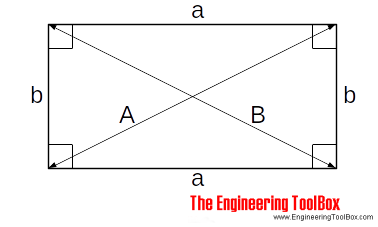Engineering ToolBox - Resources, Tools and Basic Information for Engineering and Design of Technical Applications!

# Squaring with Diagonal Measurements

## A rectangle is square if the lengths of both diagonals are equal.A rectangle where both lengths and both widths are equal is square if length of both diagonals are equal!

A = B                                  (1)

where

A, B = diagonal measurements (m, ft ..)

## Related Topics

• ### Mathematics

Mathematical rules and laws - numbers, areas, volumes, exponents, trigonometric functions and more.

## Related Documents

• ### Angle Calculator - Carpenter's Square

Calculate angles with a straight board across carpenter's square.
• ### Area Survey App

Online app that can be used to make an exact plot of a surveyed area - like a room, a property or any 2D shape.
• ### Circle - the Chord Lengths when Divided in to Equal Segments

Calculate chord lengths when dividing the circumference of a circle into an equal number of segments.
• ### Circles Outside a Circle

Calculate the numbers of circles on the outside of an inner circle - like the geometry of rollers on a shaft.
• ### Circles within a Rectangle - Calculator

Calculate the maximum number of circles within a rectangle - can be used to calculate the numbers of pipes or wires in a conduit or similar.
• ### Equal Areas - Circles vs. Squares

Radius and side lengths of equal areas, circles and squares.
• ### Geometric Shapes - Areas

Areas, diagonals and more - of geometric figures like rectangles, triangles, trapezoids ..
• ### Law of Sines

Calculate the angles in a generic triangle.
• ### Law of Tangents

Triangles and law of tangents.
• ### Smaller Circles within a Large Circle - Calculator

Calculate the number of small circles that fits into an outer larger circle - ex. how many pipes or wires fits into a larger pipe or conduit.
• ### Smaller Rectangles within a Larger Rectangle

The maximum number of smaller rectangles - or squares - within a larger rectangle (or square).
• ### Solids - Volumes and Surfaces

The volume and surface of solids like rectangular prism, cylinders, pyramids, cones and spheres - online calculator.
• ### Trigonometric Functions

Sine, cosine and tangent - the natural trigonometric functions.

## Engineering ToolBox - SketchUp Extension - Online 3D modeling!

Add standard and customized parametric components - like flange beams, lumbers, piping, stairs and more - to your Sketchup model with the Engineering ToolBox - SketchUp Extension - enabled for use with older versions of the amazing SketchUp Make and the newer "up to date" SketchUp Pro . Add the Engineering ToolBox extension to your SketchUp Make/Pro from the Extension Warehouse !

We don't collect information from our users. More about

## Citation

• The Engineering ToolBox (2010). Squaring with Diagonal Measurements. [online] Available at: https://www.engineeringtoolbox.com/squaring-diagonal-measurement-d_1714.html [Accessed Day Month Year].

Modify the access date according your visit.

9.19.12